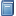Buchempfehlung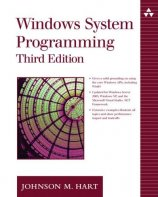Windows System Programming
Das Kompendium liefert viele interessante Informationen zur Windows-Programmierung auf Englisch. [Mehr Infos...]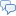FreeBASIC-Chat
Es sind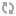Benutzer im FreeBASIC-Chat online.
(Stand:  )

# Code-Beispiel

## Komplexe Zahlen

 Lizenz: Erster Autor: Letzte Bearbeitung: GPL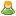nemored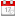15.09.2007

In der Physik und angewandten Mathematik lassen sich viele Berechnungen mit Hilfe der komplexen Zahlen einfacher ausführen. Der unten stehende Code stellt einige Funktionen zum Rechnen mit komplexen Zahlen zur Verfügung. Die Zahlen können dabei in der algebraischen Form oder in der Polarform angesprochen werden.

```type complex   as double real, imag  ' Real- und Imaginaerteil   as double abs, arg    ' Betrag und Argument end type declare function newCartesian(byval real as double, byval imag as double) as complex declare function newPolar(byval abs as double, byval arg as double) as complex declare function complexAdd(byval c1 as complex, byval c2 as complex) as complex declare function complexSub(byval c1 as complex, byval c2 as complex) as complex declare function complexMult(byval c1 as complex, byval c2 as complex) as complex declare function complexDiv(byval c1 as complex, byval c2 as complex) as complex declare function complexConj(byval c as complex) as complex declare function complexReci(byval c as complex) as complex declare sub polar2cartesian(byref c as complex) declare sub cartesian2polar(byref c as complex) declare function strCartesian overload (byval c as complex) as string declare function strCartesian(byval c as complex, byval p as ubyte) as string declare function strPolar overload (byval c as complex) as string declare function strPolar(byval c as complex, byval p as ubyte) as string function newCartesian(byval real as double, byval imag as double) as complex   ' neue komplexe Zahl anhand von Real- und Imaginaerteil definieren   dim as complex ret   ret.real = real   ret.imag = imag   cartesian2polar(ret)   return ret end function function newPolar(byval r as double, byval phi as double) as complex   ' neue komplexe Zahl anhand der Polarkoordinaten definieren   dim as complex ret   ret.abs = r   ret.arg = phi   polar2cartesian(ret)   return ret end function function complexAdd(byval c1 as complex, byval c2 as complex) as complex   ' addiert zwei komplexe Zahlen   dim as complex ret   ret.real = c1.real + c2.real   ret.imag = c1.imag + c2.imag   cartesian2polar(ret)   return ret end function function complexSub(byval c1 as complex, byval c2 as complex) as complex   ' subtrahiert zwei komplexe Zahlen   dim as complex ret   ret.real = c1.real - c2.real   ret.imag = c1.imag - c2.imag   cartesian2polar(ret)   return ret end function function complexMult(byval c1 as complex, byval c2 as complex) as complex   ' multipliziert zwei komplexe Zahlen   dim as complex ret   ret.abs = c1.abs * c2.abs   ret.arg = c1.arg + c2.arg   polar2cartesian(ret)   return ret end function function complexDiv(byval c1 as complex, byval c2 as complex) as complex   ' dividiert zwei komplexe Zahlen   dim as complex ret   ret.abs = c1.abs / c2.abs   ret.arg = c1.arg - c2.arg   polar2cartesian(ret)   return ret end function function complexConj(byval c as complex) as complex   ' bildet die konjugiert komplexe Zahl   dim as complex ret   ret = newCartesian(c.real, -c.imag)   cartesian2polar(ret)   return ret end function function complexReci(byval c as complex) as complex   dim ret as complex   if c.real=0 and c.imag=0 then     ret = newCartesian(0, 0)   else     ret = newCartesian(c.real/c.abs^2, -c.imag/c.abs^2)   end if   cartesian2polar(ret)   return ret end function sub polar2cartesian(byref c as complex)   ' ermittelt Real- und Imaginaerteil, wenn die Polarkoordinaten bekannt sind   dim as complex ret   c.real = c.abs * cos(c.arg)   c.imag = c.abs * sin(c.arg) end sub sub cartesian2polar(byref c as complex)   ' ermittelt die Polarkoordinaten, wenn Real- und Imaginaerteil bekannt sind   dim as complex ret   c.abs = sqr(c.real^2 + c.imag^2)   if c.abs = 0 then     c.arg = -1   elseif c.imag >= 0 then     c.arg = acos(c.real/c.abs)   else     c.arg = -acos(c.real/c.abs)   end if end sub function strCartesian(byval c as complex) as string   return strCartesian(c, 8) end function function strCartesian(byval c as complex, byval p as ubyte) as string   ' gibt die komplexe Zahl in der Form a+bi zurueck (gerundet auf p Stellen)   dim as string ret   ret = str(int(c.real*10^p+.5)/10^p)   if c.imag > 0 then ret += "+"   if c.imag <> 0 then ret += str(int(c.imag*10^p+.5)/10^p) + "i"   return ret end function function strPolar(byval c as complex) as string   return strPolar(c, 8) end function function strPolar(byval c as complex, byval p as ubyte) as string   ' gibt die komplexe Zahl in der Form rE(phi) zurueck   dim as string ret   ret = str(int(c.abs*10^p+.5)/10^p)   if c.arg<>0 and c.abs<>0 then ret += "E(" + str(int(c.arg*10^p+.5)/10^p) + ")"   return ret end function```

Einfache Rechenbeispiele:

```dim as complex c, d c = newCartesian(5, 7)   ' c = 5 + 7i d = newCartesian(3, -4)  ' d = 2 - 4i ' Addition; Ausgabe in algebraischer Form print strCartesian(c); " + "; strCartesian(d); print " = "; strCartesian(complexAdd(c, d)) ' Multiplikation; Ausgabe in Polarform (4 Nachkommastellen) print strPolar(c, 4); " * "; strPolar(d, 4); print " = "; strPolar(complexMult(c, d), 4) 'reziproker Wert print "1 / ("; strCartesian(d); print ") = "; strCartesian(complexReci(d)) sleep```
Zusätzliche Informationen und Funktionen
• Das Code-Beispiel wurde am 15.09.2007 vonnemored angelegt.
• Die aktuellste Version wurde am 15.09.2007 vonnemored gespeichert.